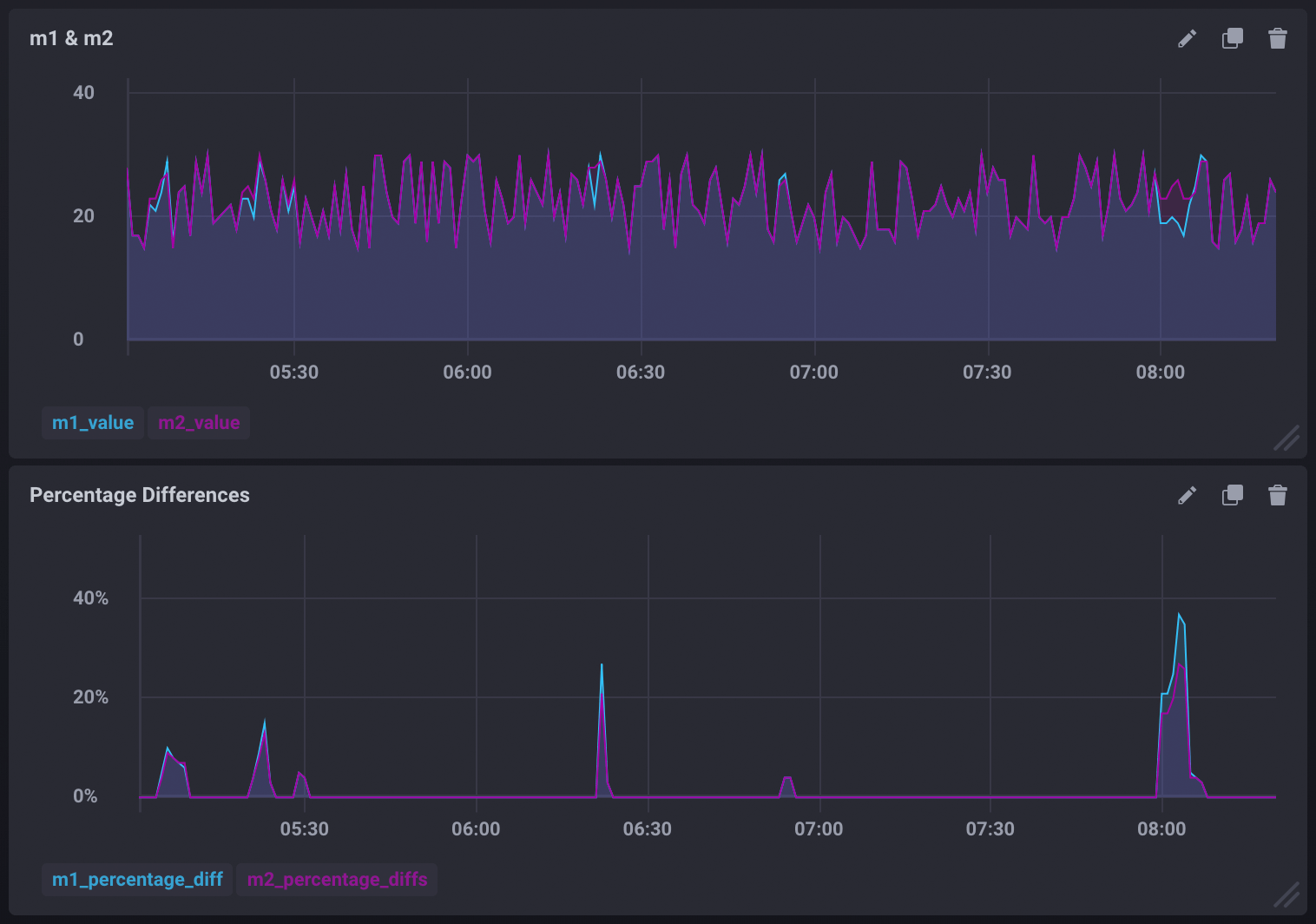# Trigger alerts by comparing two measurements

Kapacitor allows you to create alerts based on two or more measurements. In this guide, we are going to compare two measurements, `m1` and `m2`, and create an alert whenever the two measurements are different. As an added bonus, we’ll also include a query that can be used to graph the percentage difference between the two measurements.

## Comparing measurements and creating an alert

The following TICKscript streams the `m1` and `m2` measurements, joins them, compares them, and triggers an alert if the two measurements are different.

``````var window_size = 1m

// Stream m1
var m1 = stream
|from()
.measurement('m1')
|window()
.period(window_size)
.every(window_size)
.align()
|count('value')
.as('value')

// Stream m2
var m2 = stream
|from()
.measurement('m2')
|window()
.period(window_size)
.every(window_size)
.align()
|count('value')
.as('value')

// Join m1 and m2
var data = m1
|join(m2)
.as('m1', 'm2')

// Compare the joined stream and alert when m1 and m2 values are different
data
.crit(lambda: "m1.value" != "m2.value")
.message('values were not equal m1 value is {{ index .Fields "m1.value" }} m2 value is {{ index .Fields "m2.value" }}')
``````

## Graphing the percentage difference between the measurements

Use the `data` stream defined in the TICKscript above to calculate the difference between `m1` and `m2`, transform it into a float, divide that difference by the actual values of `m1` and `m2`, then multiply them by 100. This will give you the percentage difference for each. Store the difference as new fields in the `diffs` measurement:

``````data
// Calculate the difference between m1 and m2
|eval(lambda: "m1.value" - "m2.value")
.as('value_diff')
.keep()
// Calculate the % difference of m1 and m2
|eval(lambda: (float("value_diff") / float("m1.value")) * 100.0, lambda: (float("value_diff") / float("m2.value")) * 100.0)
.as('diff_percentage_m1', 'diff_percentage_m2')
// Store the calculated differences in the 'diffs' measurement
|influxDBOut()
.measurement('diffs')
.database('mydb')
.create()
``````

This can be used to create visualizations similar to:## The full TICKscript

Below is the entire, uncommented TICKscript:

``````var window_size = 1m

var m1 = stream
|from()
.measurement('m1')
|window()
.period(window_size)
.every(window_size)
.align()
|count('value')
.as('value')

var m2 = stream
|from()
.measurement('m2')
|window()
.period(window_size)
.every(window_size)
.align()
|count('value')
.as('value')

var data = m1
|join(m2)
.as('m1', 'm2')

data
.crit(lambda: "m1.value" != "m2.value")
.message('values were not equal m1 value is {{ index .Fields "m1.value" }} m2 value is {{ index .Fields "m2.value" }}')

data
|eval(lambda: "m1.value" - "m2.value")
.as('value_diff')
.keep()
|eval(lambda: (float("value_diff") / float("m1.value")) * 100.0, lambda: (float("value_diff") / float("m2.value")) * 100.0)
.as('diff_percentage_m1', 'diff_percentage_m2')
|influxDBOut()
.measurement('diffs')
.database('mydb')
.create()
``````

### Linux Package Signing Key Rotation

All signed InfluxData Linux packages have been resigned with an updated key. If using Linux, you may need to update your package configuration to continue to download and verify InfluxData software packages.

For more information, see the Linux Package Signing Key Rotation blog post.

### InfluxDB Cloud backed by InfluxDB IOx

All InfluxDB Cloud organizations created on or after January 31, 2023 are backed by the new InfluxDB IOx storage engine. Check the right column of your InfluxDB Cloud organization homepage to see which InfluxDB storage engine you’re using.

### InfluxDB Cloud backed by InfluxDB TSM

All InfluxDB Cloud organizations created on or after January 31, 2023 are backed by the new InfluxDB IOx storage engine which enables nearly unlimited series cardinality and SQL query support. Check the right column of your InfluxDB Cloud organization homepage to see which InfluxDB storage engine you’re using.